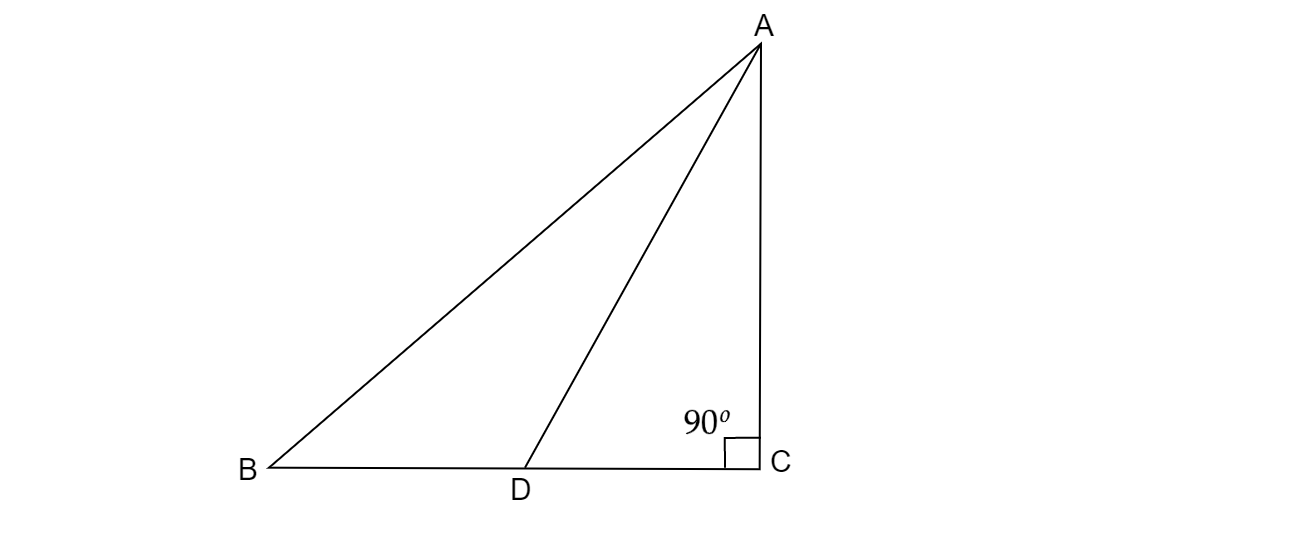"
">

# In fig. 3, ABC is a right triangle, right angled at C and D is the mid-point of BC, Prove that $( AB)^{2} =4( AD)^{2} -3( AC)^{2} .$"

Given: ABC is a right triangle, right angled at C and D is the mid-point of BC.

To do: To Prove that $( AB)^{2} =4( AD)^{2} -3( AC)^{2} .$

Solution:
Here given $AC\bot BC$                 $( \because \vartriangle ABC\ is\ a\ right\ triangle)$$BD=CD$                                       $( \because \ D\ is\ the\ mid-point\ of\ BC)$

In $\vartriangle$ABC,

$( Using Pythagoras\ theorem)$

$( AB)^{2} =( BC)^{2} +( AC)^{2}$

$\Rightarrow ( AB)^{2} =( BD+CD)^{2} +( AC)^{2}$          $( \because \ BD+CD=BC)$

$\Rightarrow ( AB)^{2} =( CD+CD)^{2} +( AC)^{2}$

$\Rightarrow ( AB)^{2} =4( CD)^{2} +( AC)^{2}$                           $\dotsc \dotsc \dotsc \dotsc \dotsc \dotsc \dotsc ( 1)$

In triangle $\vartriangle ADC$

$( On\ using\ pythagoras\ theorem)$

$( AD)^{2} =( AC)^{2} +( CD)^{2}$                                     $( \because \vartriangle ADC\ is\ also\ a\ right\ triangle)$

$\Rightarrow ( CD)^{2} =( AD)^{2} -( AC)^{2}$

$After\ substituting\ the\ value\ of\ ( CD)^{2} \ in\ equation\ ( 1)$

$\Rightarrow ( AB)^{2} =4\left(( AD)^{2} -( AC)^{2}\right) +( AC)^{2}$

$\Rightarrow ( AB)^{2} =4( AD)^{2} -4( AC)^{2} +( AC)^{2}$

$\Rightarrow ( AB)^{2} =4( AD)^{2} -3( AC)^{2}$

Hence proved.

Updated on: 10-Oct-2022

34 Views